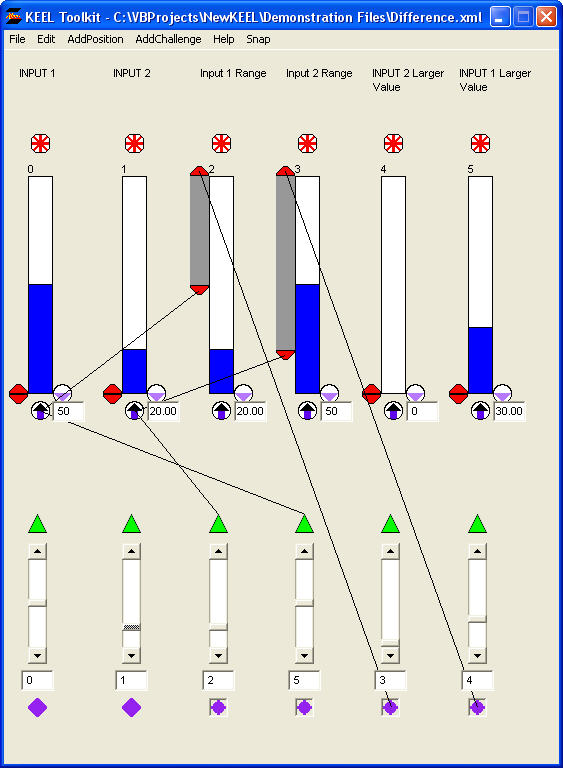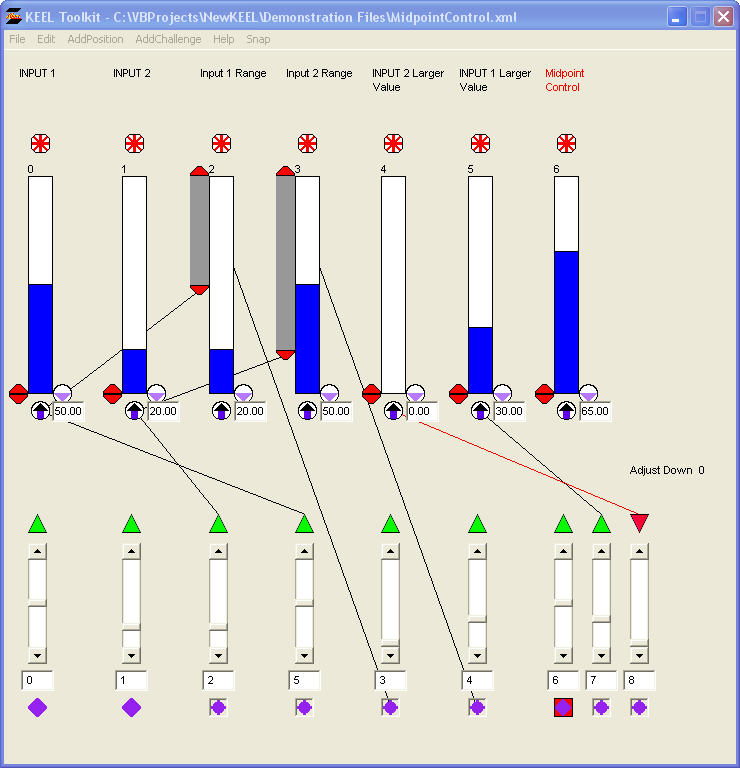# Difference between two values

Difference.xml

## Design

There may be times when one needs to have the difference between two values incorporated into a design.

NOTE: Normally mathematical functions like this would be handled external to the KEEL Engine.

For example if one has two inputs (Input_1 and Input_2), where Input_1 is 50 and Input_2 is 20 and the difference (30 in this case) is used to control another segment of the design.One example where this concept might be applied is in the case where the difference drives a deviation from a midpoint.

In the following design input (index 6) is locked at 50 (the midpoint). If 'Input 1' is larger then 'Input 2' (as shown in the screenshot below) then the difference value (20 in this case) is added as a driver to the output 'Midpoint Control'. If 'Input 2' had been larger than 'Input 1', then the difference would have been used to block the 'Midpoint Control'.

MidpointControl.xml